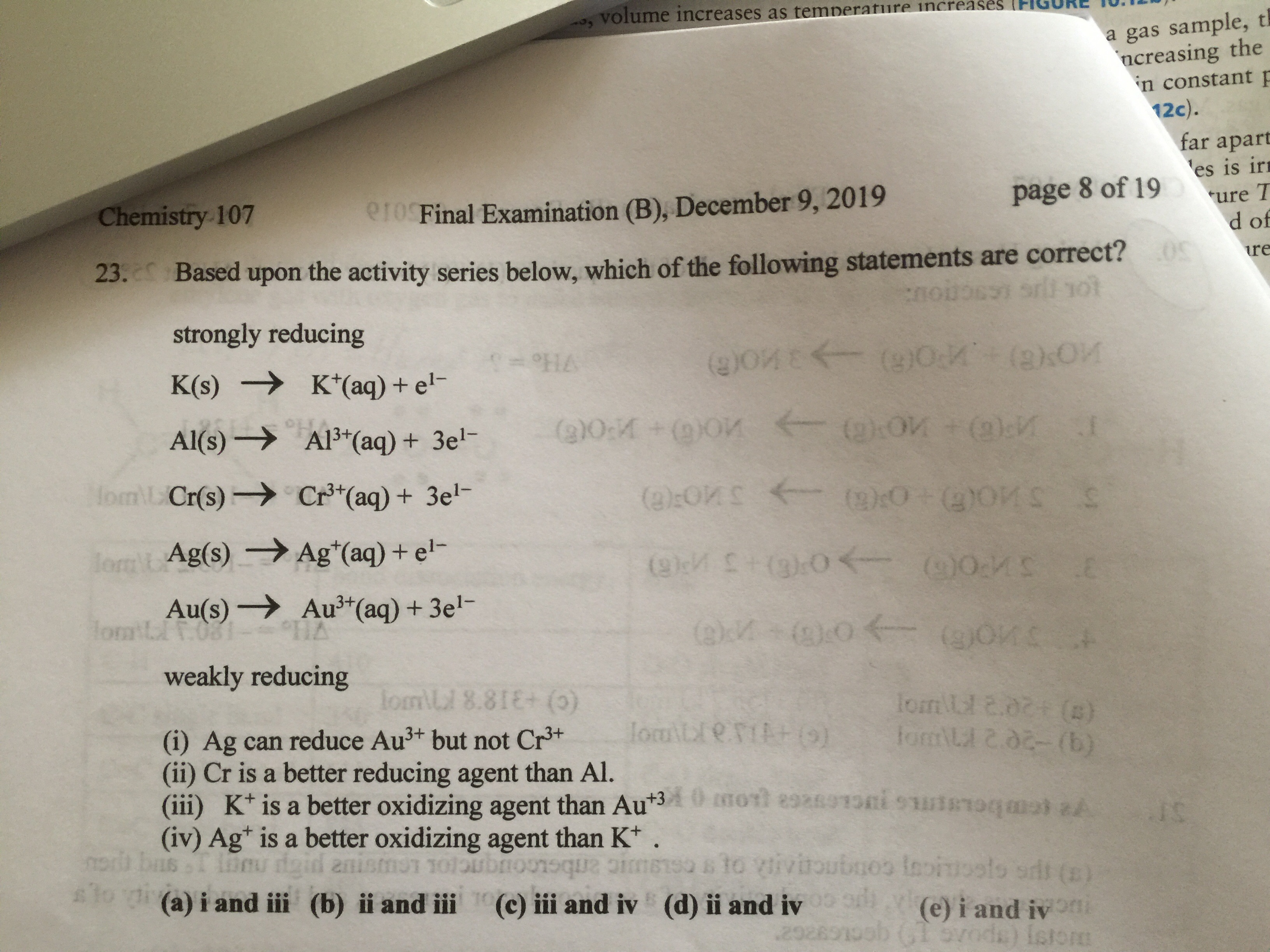# Based upon the activity series below, which of the following statements are correct? Strongly Reducing K(s) → K+(aq) + e- Al(s) → Al3+(aq) + 3 e- Cr(s) → Cr3+(aq) + 3 e- Ag(s) → Ag+(aq) + e- Au(s) → Au3+(aq) + 3 e- Weakly Reducing (i) Ag can reduce Au3+ but not Cr3+. (ii) Cr is a better reducing agent than Al. (iii) K+ is a better oxidizing agent than Au3+. (iv) Ag+ is a better oxidizing agent than K+.